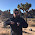## Wednesday, January 21, 2015

### Is this the market monetarist model?

Scott Sumner wrote down a "model"

$$\text{(1) } H_{t+1} - H^{n}_{t} = \alpha (NGDP_{t} - NGDP^{T}_{t})$$

$$\text{(2) } NGDP_{t} = NGDP^{F}_{t-1} + e_{t}$$

$$\text{(3) } NGDP^{F}_{t-1} =NGDP^{T}_{t} + SE_{t-1}$$

Where $H$ is the number of hours worked, $H^{n}$ is the "natural rate" of hours worked, the superscript $T$ means the central bank's target, $F$ is the futures market and $SE$ is a 'systematic error' (from measuring the difference between the futures market NGDP and the central bank's target NGDP).

This "model" does nothing except assert Scott Sumner's view of economics. As such, it carries no real weight. It's a bit like saying a translation of Sumner's blog into Italian is an Italian model. Che fa?! According to equation (2), NGDP in period $t$ is the futures market NGDP in period $t-1$ (an NGDP futures market forecasts NGDP as well as it can possibly be forecast). Or, if the central bank is excellent at forecasting NGDP, then the "systematic error" $SE$ is zero (or just random, like $e_{t}$) and if it isn't, it's not. That's really the entire content of equation (3).

Besides, it isn't clear that futures markets actually work.

However, equation (1) is interesting, because, well, it's almost an information transformation model. If we look at the model $P: NGDP \rightarrow H$, we can write (like the labor market model here)

$$P = \alpha \frac{NGDP}{H}$$

The best fit (using CPI inflation, all items) give us an ok model of the price level and a fairly good model of the inflation rate (it is systematically high):

The key to understanding this is that we can rewrite the previous equation and take to derivative to obtain another version of Okun's law

$$H = \alpha \frac{NGDP}{P}$$

$$\frac{d}{dt} \log H = \frac{d}{dt} \log \frac{NGDP}{P} + \frac{d}{dt} \log \alpha$$

$$\frac{d}{dt} \log H = \frac{d}{dt} \log RGDP$$

According to Okun's law, RGDP growth is correlated with the change in the employment rate (anticorrelated with the change in the unemployment rate), along with anything else that roughly measures the quantity of employment (like total hours worked).

As a log-linearized model, the information transfer version of Sumner's equation (1) is

$$h_{t} = n_{t} - \pi_{t} + c_{h}$$

Which means that the natural rate of hours worked and the central bank target NGDP in Sumner's model must basically combine to give you the price level:

$$H^{n} - \alpha NGDP^{T} \sim P$$

What gives the information transfer model version  some weight relative to Sumner's equation (1) is that it follows from information equilibrium -- information in the signals from the aggregate demand are received by labor hours (and the price level detects the information transfer). Also, Sumner's model doesn't tell us the relationship between the his central bank NGDP target and the natural rate of hours worked (I am unsure if he allows them to be related as one is a fundamental property of the economy and the other is chosen by the central bank).

Sumner has complained that I don't have a story behind my theory, however he doesn't seem to have any theory behind his story.

1.Regarding futures markets working, I saw this the other day...

http://monetaryrealism.com/scott-sumner-has-a-new-job/

1.I saw that -- however Sumner does have solutions for some of the specific problems (especially the liquidity) that Sankowski brings up. In particular, Sumner's idea is not for just setting up a place for an NGDP futures market (I'm not sure Sankowski gets the designs right in his list of 1-5), but rather a subsidized market. Sumner thinks the Fed should put up billions of dollars that can be won by the purchasers of futures market contracts -- which I don't think is any of the list of 1-5.

In theory, people will always be incentivized to get the right answer because they win money if they do and lose if they don't; it doesn't matter if they don't care about NGDP (or don't have a stake in it).

The future market design is actually a bit like more like Intrade (and similar to what Sumner is trying to do now). However, besides the problems I point to here:

http://informationtransfereconomics.blogspot.com/2015/01/is-market-intelligent.html

... there is reality that Nate Silver and Sam Wang did as well as prediction markets using polls -- you could probably get a forecast as good as an NGDP futures market using polls as well. Markets seem to be asynchronous polls, at least for the information aggregation problem. But people actually vote so asking them how they will vote should give you some insight; people don't decide which economic shocks happen, so polling gets you stuff like this:

http://informationtransfereconomics.blogspot.com/2015/01/better-than-fed.html

... which is ostensibly a poll of the people who make NGDP happen (at least according to Sumner).

2.... thanks for the review Jason.

Comments are welcome. Please see the Moderation and comment policy.

Also, try to avoid the use of dollar signs as they interfere with my setup of mathjax. I left it set up that way because I think this is funny for an economics blog. You can use € or £ instead.